Light waves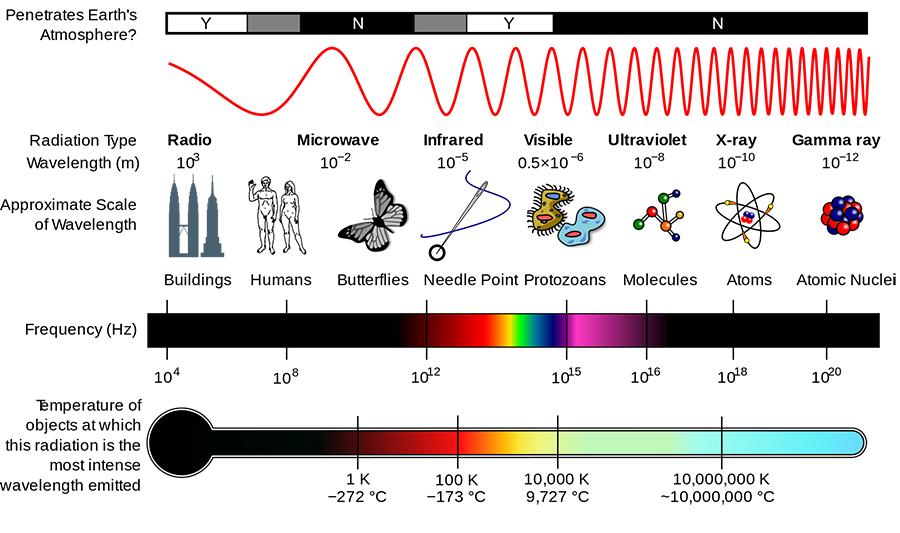Image source

Light waves are electromagnetic radiation, with a very wide range of wavelengths. Visible light makes up a very small portion of the electromagnetic spectrum.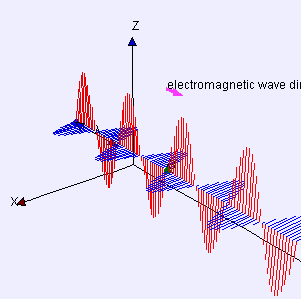Light waves can travel in vacuum, which means they do not rely on the motion of particles in a medium, like mechanical waves. The electric and magnetic components of the wave are generally perpendicular, and peak at the same time. The strength of the electromagnetic field oscillates as the wave propagates. A charged particle will oscillate in response to a passing electromagnetic wave.

Light is the signal passing between charged particles. In a sense, it is the way electrons "talk" to each other. An accelerating charged particle emits light waves.

Radio waves are long-wavelength light waves. Oscillating electrons in a transmitter emit light waves that are "felt" by other electrons in a receiver. The receiving electrons oscillate due to the changing electromagnetic field.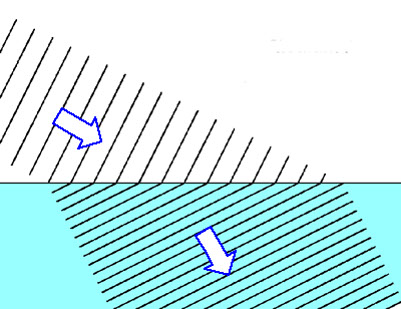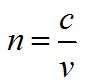Light waves travel at a constant speed in vacuum, regardless of the motion of the observer. The speed of light in vacuum is about 3.0 x 108 m/s, and is generally denoted as c. In a medium, the photons of light (light particles) interact with matter particles. Light still travels at c when it is propagating as a wave, but photons of light are absorbed and re-emitted by the matter particles, which takes time. This means that the effective speed of light in a medium is slower than c.

We define the index of refraction n, as the ratio of the speed of light in vacuum to its effective speed in a material. The index of refraction is a property of the material. The frequency of light stays constant as the light passes through materials of varying n, and the wavelength changes according to the effective speed v.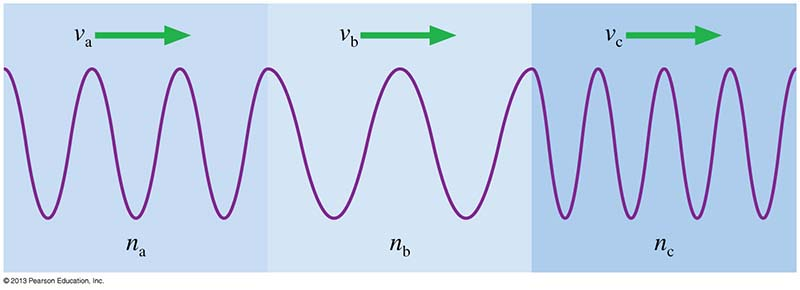This illustration shows a snapshot in time of a wave traveling through media with different indices of refraction. Which index of refraction is the largest?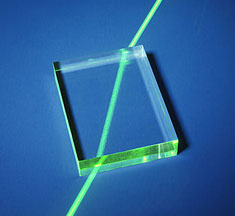In vacuum, the index of refraction is 1. Air has a very low index of refraction, still about 1.0. The higher the index of refraction, the slower the effective speed of light in the medium. Glass has an index of refraction of about 1.5.

Practice problem: Orange light with a wavelength of 600 nm is propagating through air. The light ray strikes a glass slide 1.00 mm thick, perpendicular to its surface, and propagates through the glass. How many wavelengths of light are inside the glass slide?